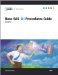# List of Figures

## Chapter 1: The CORR Procedure

Figure 1.1: Univariate Statistics
Figure 1.2: Pearson Correlation Coefficients
Figure 1.3: Symmetric Matrix Plot (Experimental)
Figure 1.4: Correlations between Two Variables

## Chapter 2: The FREQ Procedure

Figure 2.1: Crosstabulation Table
Figure 2.2: Statistics Produced with the CHISQ Option
Figure 2.3: Crosstabulation Table and Statistics for Boys
Figure 2.4: Crosstabulation Table and Statistics for Girls
Figure 2.5: Test for the Hypothesis of No Association
Figure 2.6: Agreement Study
Figure 2.7: Missing Values in Frequency Tables

## Chapter 3: The UNIVARIATE Procedure

Figure 3.1: Basic Measures and Extreme Observations
Figure 3.2: Histogram for Loan-to-Value Ratio
Figure 3.3: Quantiles for Loan-to-Value Ratio
Figure 3.4: Comparative Histogram for Loan-to-Value Ratio
Figure 3.5: Moments and Tests for Normality
Figure 3.6: Normal Probability Plot
Figure 3.7: Compass Positions for Inset
Figure 3.8: Coordinate Position for Inset
Figure 3.9: Reference Point for Inset
Figure 3.10: Construction of a Q-Q PlotBase SAS 9.1 Procedures Guide, Volumes 1, 2, 3 and 4
ISBN: 1590472047
EAN: 2147483647
Year: 2004
Pages: 74